Question
For the year 2017, a company reports GAAP(accrual basis) net income of \$6 million. Depreciation expense was \$200,000. From 2016 to 2017 current assets decreased by \$400,000, while current liabilities decreased by \$200,000. Using indirect method what is the cash flow from operations?

 Net Income 6,000,000 Depreciation expense 200,000 Decrease in current assets 400,000 Decrease in current liabilities -200,000 Net Cash Flows from Operations \$6,400,000

#### Earn Coins

Coins can be redeemed for fabulous gifts.

Similar Homework Help Questions
• ### Blossom Company reported net income of \$3.3 million in 2017. Depreciation for the year was \$169,200,...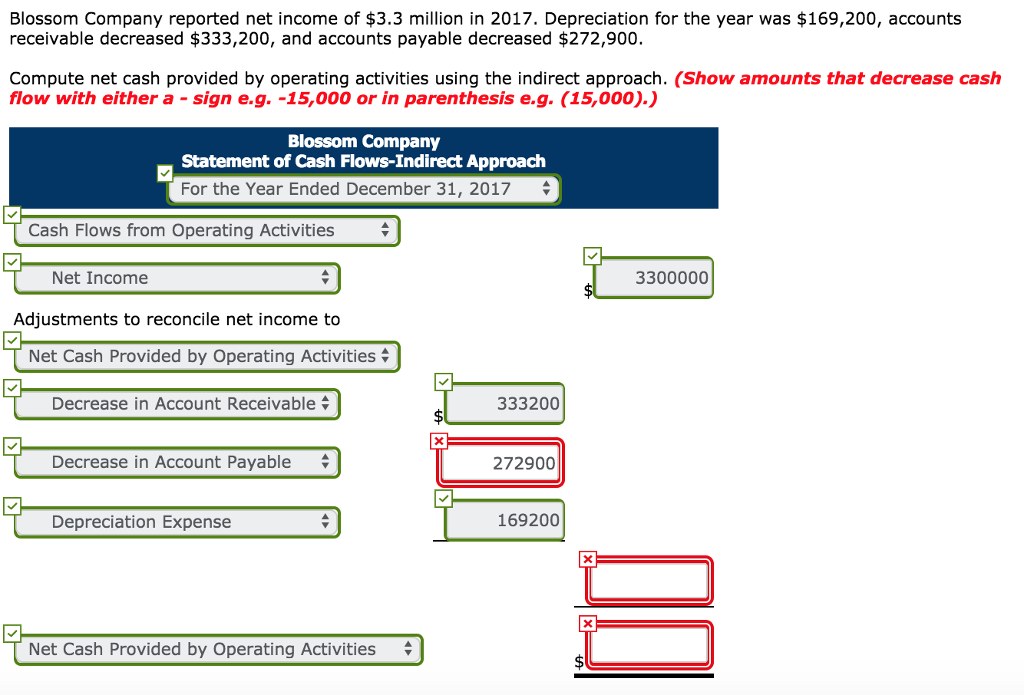Blossom Company reported net income of \$3.3 million in 2017. Depreciation for the year was \$169,200, accounts receivable decreased \$333,200, and accounts payable decreased \$272,900. Compute net cash provided by operating activities using the indirect approach. (Show amounts that decrease cash flow with either a sign e.g. -15,000 or in parenthesis e.g. (15,000).) Blossom Company Statement of Cash Flows-Indirect Approach For the Year Ended December 31, 2017 Cash Flows from Operating Activities * Net Income 3300000 Adjustments to reconcile net...

• ### The Castillo Company reported operating profits of \$60,000, Depreciation expenses of \$40,000, Interest expense of \$10,000...

The Castillo Company reported operating profits of \$60,000, Depreciation expenses of \$40,000, Interest expense of \$10,000 and paid \$10,000 in taxes in 2017. Following are the Company’s balance sheets for 2016 and 2017. C&B CASTILLO COMPANY                                                             2016                    2017 Cash                                                    \$50,000              \$5,000 Accounts Receivables                         200,000           300,000    Inventories                                          400,000           480,000 Total Current Assets                         650,000           785,000 Gross Fixed Assets                             450,000           570,000 Accumulated Depreciation                -100,000           -140,000 Net Fixed Assets                                ...

• ### QUESTION 1 A company had net income of \$252.000. Depreciation expense was \$26,000. During the year,...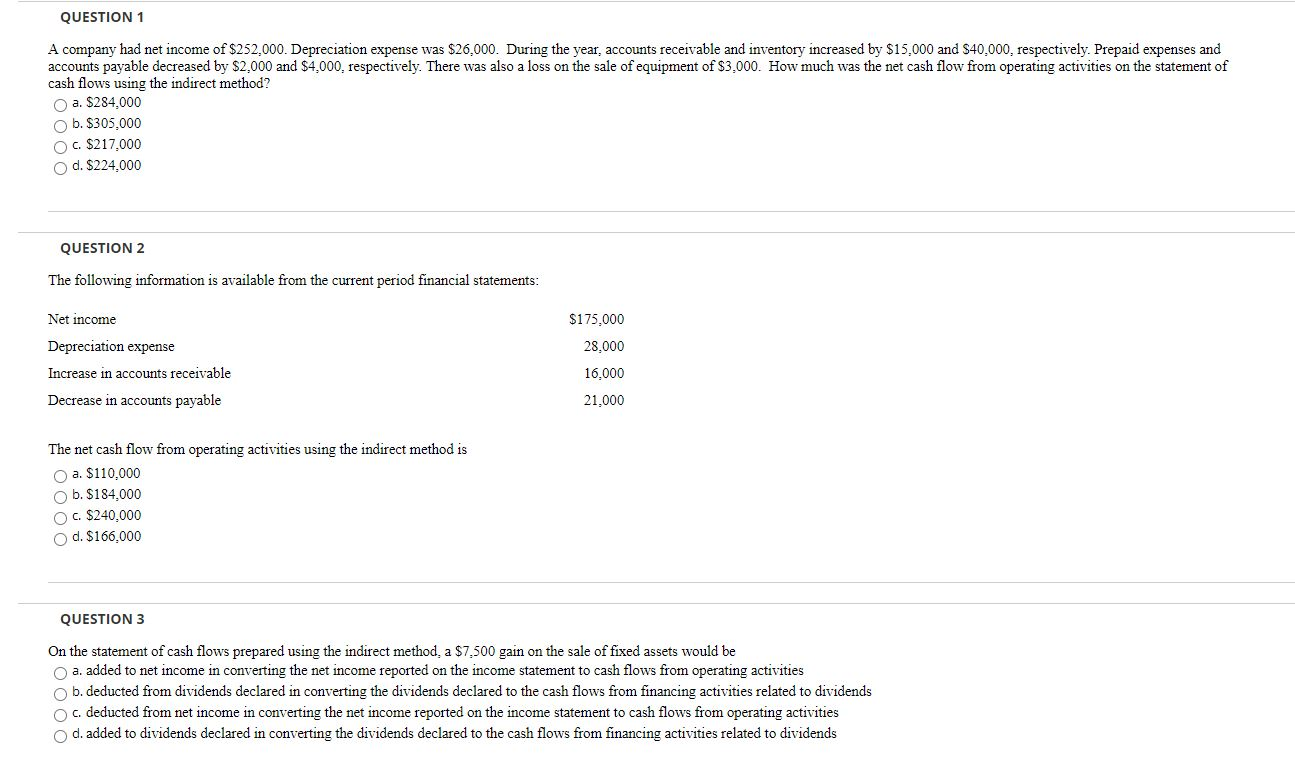QUESTION 1 A company had net income of \$252.000. Depreciation expense was \$26,000. During the year, accounts receivable and inventory increased by \$15.000 and \$40.000, respectively. Prepaid expenses and accounts payable decreased by \$2.000 and \$4,000, respectively. There was also a loss on the sale of equipment of \$3,000. How much was the net cash flow from operating activities on the statement of cash flows using the indirect method? a. \$284.000 b. \$305,000 c. \$217,000 d. \$224.000 QUESTION 2 The...

• ### Wildcat Company has an accrual basis net income of \$175,000 and the following related items: Depreciation...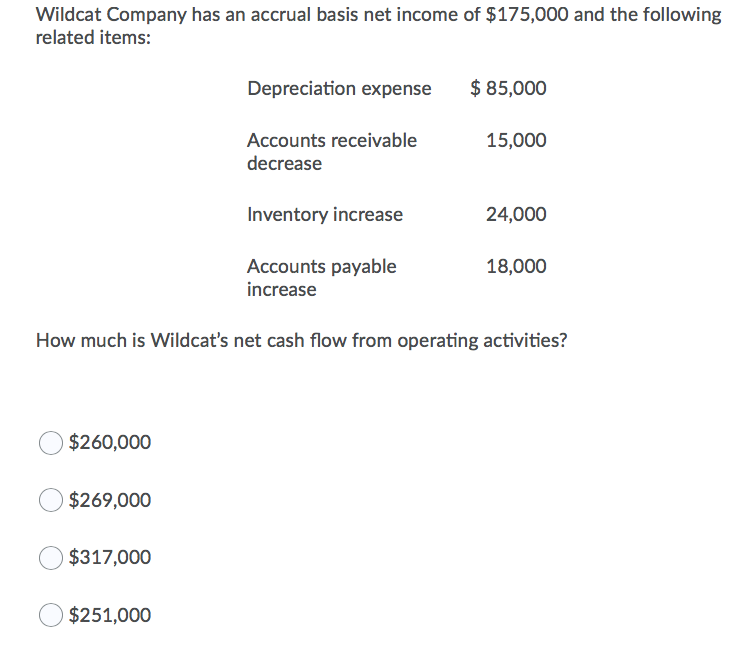Wildcat Company has an accrual basis net income of \$175,000 and the following related items: Depreciation expense \$ 85,000 15,000 Accounts receivable decrease Inventory increase 24,000 18,000 Accounts payable increase How much is Wildcat's net cash flow from operating activities? \$260,000 \$269,000 \$317,000 \$251,000

• ### Brief Exercise 13-5 The net income for Metz Co. for 2017 was \$264,300. For 2017, depreciation...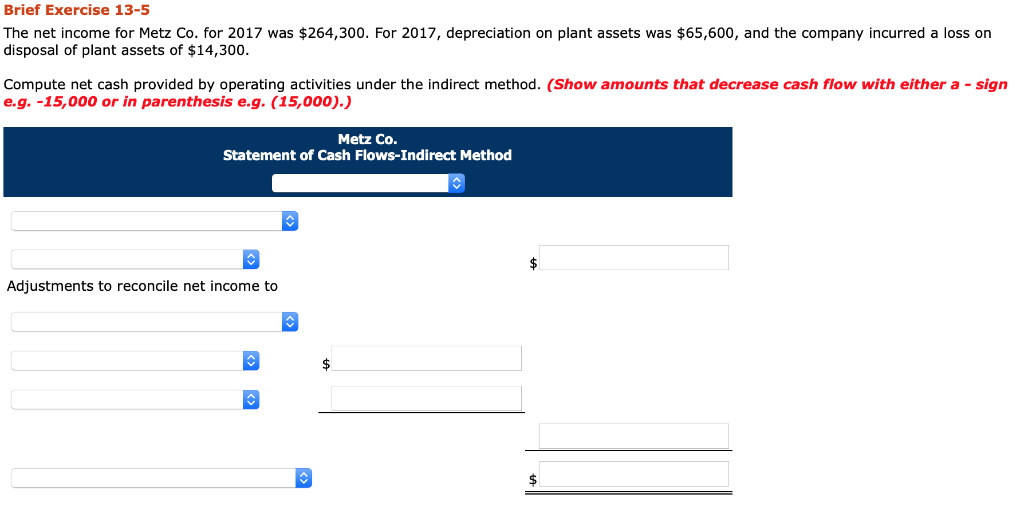Brief Exercise 13-5 The net income for Metz Co. for 2017 was \$264,300. For 2017, depreciation on plant assets was \$65,600, and the company incurred a loss on disposal of plant assets of \$14,300. Compute net cash provided by operating activities under the indirect method. (Show amounts that decrease cash flow with either a - sign e.g. -15,000 or in parenthesis e.g. (15,000).) Metz Co. Statement of Cash Flows-Indirect Method \$ Adjustments to reconcile net income to <> Brief Exercise...

• ### Assume that Galena Company's income statement showed depreciation expense of \$10,000, a on sale of investments...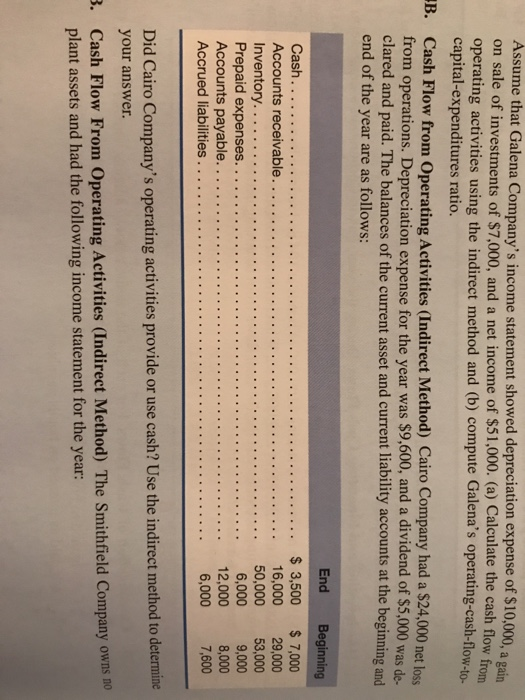Assume that Galena Company's income statement showed depreciation expense of \$10,000, a on sale of investments of \$7,000, and a net income of \$51,000. (a) Calculate the cash flow from operating activities using the indirect method and (b) compute Galena's operating-cash-flovw capital-expenditures ratio. gain ow-to- B Cash Flow from Operating Activities (Indirect Method) Cairo Company had a \$24,000 net loss from operations. Depreciation expense for the year was \$9,600, and a dividend of \$5,000 was de. clared and paid. The...

• ### 10 A company had net income of \$231,467. Depreciation expense was \$26,338. During the year, accounts...

10 A company had net income of \$231,467. Depreciation expense was \$26,338. During the year, accounts receivable and inventory increased by \$18,957 and \$38,824, respectively. Prepaid expenses and accounts payable decreased by \$1,764 and \$5,616, respectively. There was also a loss on the sale of equipment of \$4,598. How much was the net cash flow from operating activities on the statement of cash flows using the indirect method? a.\$200,770 b.\$246,388 c.\$262,403 d.\$191,574 11 Land costing \$130,147 was sold for \$174,490...

• ### A company had net income of \$261,141. Depreciation expense was \$29,049. During the year, accounts receivable...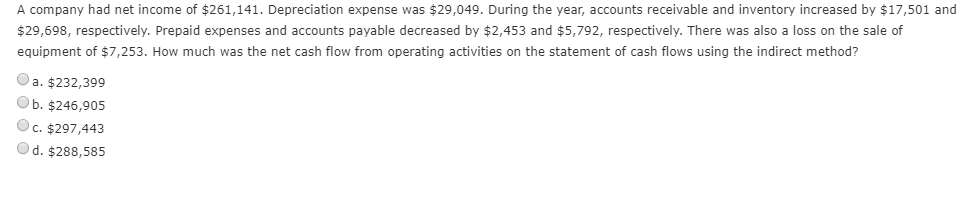A company had net income of \$261,141. Depreciation expense was \$29,049. During the year, accounts receivable and inventory increased by \$17,501 and \$29,698, respectively. Prepaid expenses and accounts payable decreased by \$2,453 and \$5,792, respectively. There was also a loss on the sale of equipment of \$7,253. How much was the net cash flow from operating activities on the statement of cash flows using the indirect method? a. \$232,399 b. \$246,905 c. \$297,443 O d. \$288,585

• ### Blossom Company reported net income of \$ 173.510 for 2017.Blossom Company also reported depreciation expense of...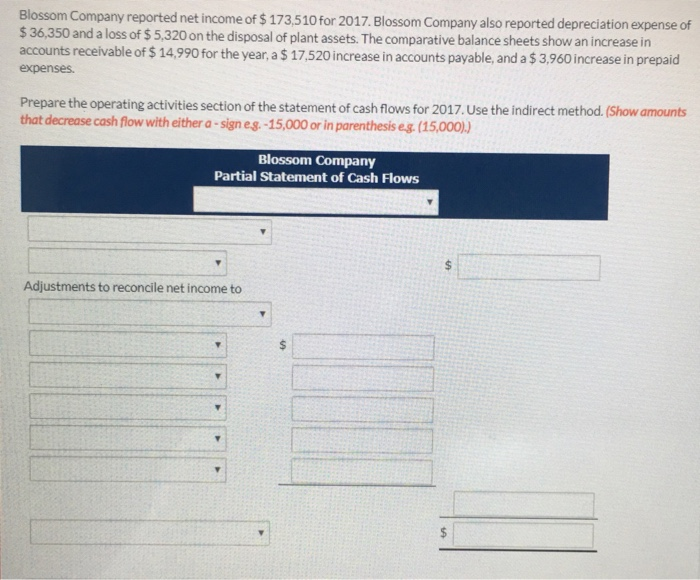Blossom Company reported net income of \$ 173.510 for 2017.Blossom Company also reported depreciation expense of \$36,350 and a loss of \$ 5,320 on the disposal of plant assets.The comparative balance sheets show an increase in accounts receivable of \$ 14,990 for the year, a \$ 17,520increase in accounts payable, and a \$ 3,960 increase in prepaid expenses. Prepare the operating activities section of the statement of cash flows for 2017. Use the indirect method. (Show amounts that decrease cash...

• ### Express Corporation had a net income of \$66,000 during 2011. During the year, the company had...

Express Corporation had a net income of \$66,000 during 2011. During the year, the company had depreciation expense of \$28,000. Accounts receivable decreased by \$22,000, and accounts payable decreased by \$10,000. Those were the company’s only current assets and liabilities. Use the indirect method to determine net cash flows from operating activities.